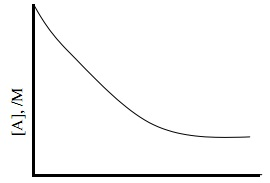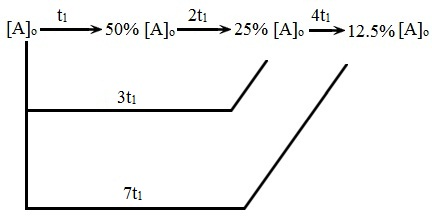#### First and Second Order Reactions, Chemistry tutorial

Integrated Rate Law for First Order Reactions:

Let us take the given reaction that is experimentally found to be of first order:

A → Product

Applying the equation that is, Reaction rate = k [Reactant]n we can represent:

Rate = -d[A]/dt = k[A]1

Here 'k' is the first order rate constant and n = 1. This signifies that the rate of consumption of A at any specified time is directly proportional to the first power of the concentration of A at that particular time.

In order to acquire the integrated rate for first order reaction, concentrations of A at the starting of the reaction and at a time t as illustrated below:

At time = 0 (that is, at the start), the concentration of A = [A]o

At time = A the concentration of A - [A]t

By utilizing these limits of concentration and time, we can integrate equation Rate = -d[A]/dt = k[A]1 after rearranging it as shown:

[A]o[A]t - d[A]/[A] = ot kdt = k dt

That is, - {ln[A]} = k[t] o[A]tt [A]t

- {ln[A]t - ln[A]o} = k (t - 0)

ln[A]o - ln[A]t = kt

Therefore, ln([A]o/[A]t) = kt

The equation above can be represented in the exponential form as shown:

[A]t = [A]o e-kt

Note: In order to comprehend the transformation of equation into - {ln[A]t - ln[A]o} = k (t - 0)

The transformation of ln([A]o/[A]t) = kt into [A]t = [A]o e-kt could be illustrated as shown.

ln([A]o/[A]t) = kt

-ln([A]t/[A]o) = kt

By taking the antilogarithms,

[A]t/[A]o = e-kt

[A]t = [A]o e-kt

Corresponding to the equation above, we can draw the concentration versus time plot for the first order reaction. This curve is of the kind illustrated in the figure shown below. Such a curve is termed as an exponential decay curve. In an exponential decay curve, there is an initial steep decrease in concentration; this is followed via a slow decrease in concentration. It will be noted that the decay curve goes parallel to x-axis after longer time intervals, point out that the reaction will take infinite time for completion.Fig:  Exponential Decay of first order reaction

The Radioactive decay is a good illustration of first order/reaction.

From the equation ln([A]o/[A]t) = kt, it is well-situated to work with logarithms to the base 10 (termed as common logarithms). Therefore, we can represent equation ln([A]o/[A]t) = kt as shown:

As well noted that: ln x = 2.303 log x

2.303 log ([A]o/[A]t) = kt

k = (2.303/t) log ([A]o/[A]t)

The equation above is helpful in computing the concentration of the reactant at a time t (that is, [A]t) provided; its initial concentration (that is, [A]o), k and t are acknowledged. As well 'k' can be computed if [A]0, [A]t and t are known. By using the graphical technique, we can test whether a reactant follows the first order or not.

Graphical method of calculating First order rate constant:

In order to ease graphical representation, equation k = (2.303/t) log ([A]o/[A]t) is modified as shown below:

log ([A]o/[A]t) = kt/2.303

Therefore, log [A]o - [A]t = kt/2.303

That is, - log [A]t = (kt/2.303) - log [A]o

log [A]t = - (k/2.303) t + log [A]o

From the equation log ([A]o/[A]t) = kt/2.303, we can derive log [A]o - [A]t = kt/2.303 by replacing the concentrations of A at the intervals t1 and t2 as follow:

At time = t1, the concentration of A = [A]1

At time = t2, the concentration of A = [A]2

log ([A]o/[A]1) = kt1/2.303

log ([A]o/[A]2) = kt2/2.303

Therefore:

log ([A]o/[A]1) - log ([A]o/[A]1) = (k/2.303) (t2 - t1)

That is, log [A]1/[A]2 = (k/2.303)(t2 - t1)

By comparing the equation log [A]t = - (k/2.303) t + log [A]o by the equation for a straight line,

y = mx + c,

We can conclude that by plotting log; [A] against time t, a straight line should be obtained for a first order reaction. The rate constant can be computed from the slope as:

k = - 2.303 x slope

Recognizing the concentration of a reactant undergoing first order reaction at a specific time, it is possible to compute its concentration at the other time interval by utilizing the equation below:

log [A]1/[A]2 = kt (t2 - t1)/2.303

The given hints might be helpful while computing the rate constant by using the integrated rate law.

A) If the concentrations of the reactant are provided at different time intervals, then the concentration at t = 0 is equivalent to [A]0 and the concentration at any specified time is [A]t; log [A]t against t plot is made.

B) Rather than providing the concentrations of a reactant at different time intervals, parameters like partial pressures, absorbance, volumes, titre values and so on that are proportional to the concentration of the reactant might be given. In such cases, the measurements made at zero time and at any specific time t might be employed rather than [A]d and [A]t. For illustration, we can replace the partial pressure of the reactant rather than its concentration in log ([A]o/[A]t) = kt/2.303 and acquire log ([A]o/[A]t) = kt/2.303

log [(PA)o/(PA)t] = kt/2.303

Here, (PA)0 and (PA)t are the partial pressures of the reactant at the beginning and after a time, t.

C) At times, the rates of measurement are made in terms of the concentrations of the product made. If the Stoichiometry of the reaction is such that one molecule of the product is made if one molecule of the reactant is consumed then the concentration of the product at t = ∞ must be equivalent to initial concentration of the reactant. Let us suppose that the concentration of the product at any particular time is x. Then x as well symbolizes the decrease in the concentration of the reactant after a time, t. Therefore [A]0 = concentration of the product at t = ∞ and [A]t = [A]0 - x .

By using such (relationships, Equation log [A]o - [A]t = kt/2.303 could be represented as,

log [A]o/[A]0-x = kt/2.303

Half-Life of First Order Reactions:

The time consumed for the concentration of a reactant to fall to half its initial value is known as the half-life of a reaction. It is represented by the symbol, t. We can derive an expression useful in computing the half-life of a substance undergoing the first order reaction by employing equation log ([A]o/[A]t) = kt/2.303

We should remember that if:

t = t1/2, [A]t = [A]o/2

By using such relationships in equation log ([A]o/[A]t) = kt/2.303, will provide:

log [A]o/[A]o/2 = kt1/2/2.303

That is, log 2 = kt1/2/2.303

Or t1/2 = 2.303 log 2/k = 0.693/k

From the above equation; we comprehend that t1/2 doesn't depend on initial concentration of the substance in the case of a first order reaction. This signifies that for a particular first order reaction, half-life period is similar, whatever be the initial concentration; this leads to an interesting yield that the time taken for the changes in the concentrations of the reactant like,

[A]o → [A]o/2

Or [A]o/2 → [A]o/4

Or [A]o/4 → [A]o/8

is similar. The half-life periods needed to bring about decrease in concentration of a reactant up to 12.5% of its initial concentration can be symbolized as in the figure shown below.Fig: Half-Life of First Order Reactions

Integrated Rate Laws for Second Order Reactions:

There are two kinds of second order reactions:

A) A single reactant could give mount to products via a second order reaction.

A → Products

The rate equation is:

rate = -d[A]/dt = k[A]2

Here, 'k' is the second order rate constant.

Two illustrations of this kind are illustrated below:

2NO2 (g) → 2NO (g) + O2 (g)

2HI (g) → H2 (g) + I2 (g)

B) The two different reactant molecules could react to give products via a second order reaction.

A + B → Products

The rate equation is represented as:

Reaction rate = k [A] [B]

Illustrations of this kind are given below. It will be noted that the stoichiometric ratio to the reactant is 1:1.

H2 (g) + I2 (g) → 2 HI (g)

CH3Br (aq) + OH- (aq) → CH3OH (aq) + Br- (aq)

CH3COOC2H5 (aq) + OH- (aq) → CH3COO- (aq) + C2H5OH

Again the study of such reactions could be simplified if the initial concentrations of both the reactants ([A]0 and [B]0) are similar, that is,

That is, [A]0 - [B]0 and therefore [A] = [B]

Here, [A] and - [B] are concentrations of the reactants at any specified time, t

Then, the equation Reaction rate = k [A] [B] takes the similar form as equation rate = -d[A]/dt = k[A]2

Rate = -d[A]/dt = k[A][B] = k [A][A]

= k[A]2

Therefore, we could observe that the rate equation takes the similar form for second order reaction, if the reaction is:

a) Second order in a single reactant or

b) First order in each of the two reactants in such a way that the concentrations of the two are similar all through the reaction.

Half-Life of a Second Order Reaction:

For reactions following the second order rate with respect to equation [rate = -d[A]/dt = k[A]2] an equation could be derived which is helpful in computing the  half-life period.

At half-life period (t = t1/2), [A]t - [A0]/2. By employing this in equation 1/[A]t = 1/[A]o + kt provides:

1/[A]o/2 = 1/[A]o + kt/2

2/[A]o = 1/[A]o = kt1/2Fig: Half-Life of a Second Order Reaction

t1/2 = 1/k[A]0

From the above, we understand that t1/2 is inversely proportional to the initial concentration for second order reaction. As initial concentration of the reactant reduces, t1/2 increases. If for the decrease in concentration of A to 50% of its initial value, time needed is 100s, then for the change from 50% A to 25% A, it will need 200s.

The three successive half-lives for a second order reaction can be symbolized by the figure shown above.

Tutorsglobe: A way to secure high grade in your curriculum (Online Tutoring)